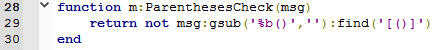# Correct number of parenthesis and ends, challenge response.

• @GoldenPhysics gave me a challenge on my post asking for challenges here. This is my solution.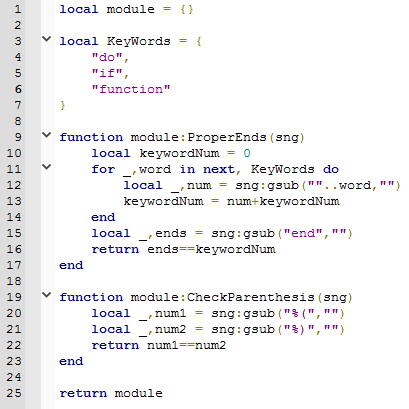This seems to work fine.

How it works:

Both solutions are simple counters. For the `CheckParenthesis` function, I could the number of ) in the string, and check if the number is the same as (. Rather simple. For the `ProperEnds` function, I check how many KeyWords are in the string, and check if it's the same as the number of ends in the string.

This seems to work well.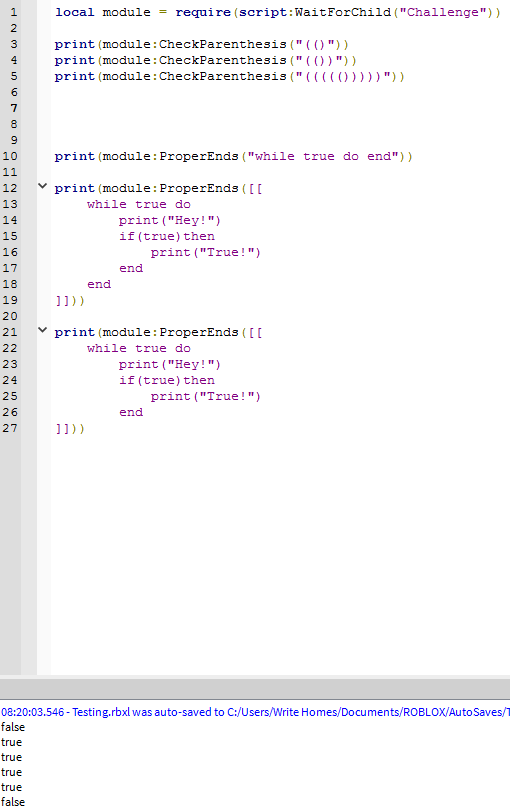Here's the code:

``````local module = {}

local KeyWords = {
"do",
"if",
"function"
}

function module:ProperEnds(sng)
local keywordNum = 0
for _,word in next, KeyWords do
local _,num = sng:gsub(word,"")
keywordNum = num+keywordNum
end
local _,ends = sng:gsub("end","")
return ends==keywordNum
end

function module:CheckParenthesis(sng)
local _,num1 = sng:gsub("%(","")
local _,num2 = sng:gsub("%)","")
return num1==num2
end

return module
``````

## Notice:

This doesn't work very well because I couldn't figure out how to properly do this. The code finds the if in elseif and counts it, which causes errors. Variables named anything with if, do, or function will count an end, and etc. I couldn't fix this problem, but I tried. I would like to see a proper solution.

• @OldPalHappy It seems like your code doesn't check for the proper order of parenthesis. I haven't actually tested this, but I would think based on your code that `())(` would return true instead of false. As for the keyword problem, I'll guess that if you make it detect at least one space before and after the keyword, it would ignore `elseif` and other variable problems.

• @GoldenPhysics Oh, I wasn't aware I had to check the order as well.

And yes, I did know that I could check to see if there weren't any characters in front of or behind the keywords, but that would require a different method. I might try again later. :3

• @GoldenPhysics Okay, I took some time to think this over, and I thought of an algorithm for the parentheses, I believe. After testing these solutions I believe they're correct as you wanted them for the challenge.

Example: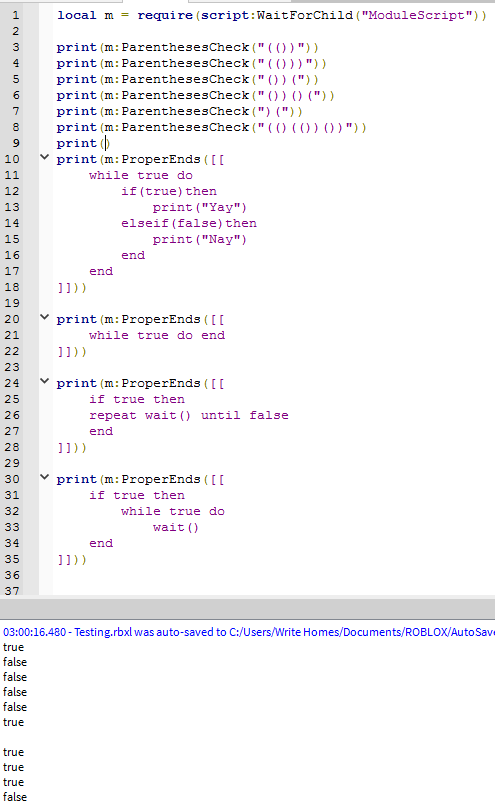Matching everything up you'll find everything works. My code isn't too long either.

Starting with the `EndsCheck`, because I was pretty close the first time, I just needed to do what you said, and check if the character in front of and behind the replaced keyword was a character.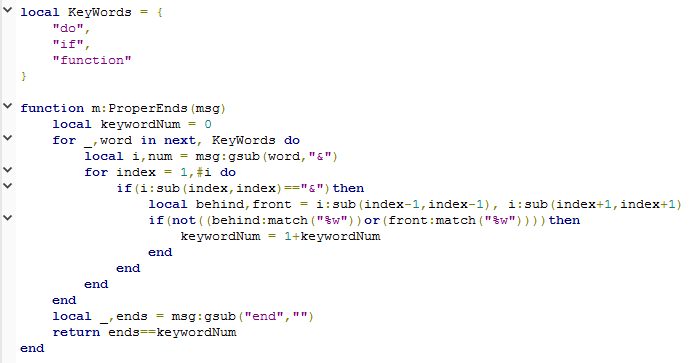After that I made the `ParenthesesCheck`. I had trouble thinking of a way to do this, but I found checking if `(` would match another `)` after the `(`, if that makes sense. Basically just matching parenthesis together. Still not sure on parentheses, or parenthesis....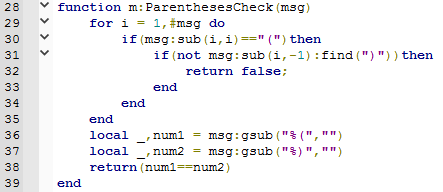Here's the code:

``````local m = {}

local KeyWords = {
"do",
"if",
"function"
}

function m:ProperEnds(msg)
local keywordNum = 0
for _,word in next, KeyWords do
local i,num = msg:gsub(word,"&")
for index = 1,#i do
if(i:sub(index,index)=="&")then
local behind,front = i:sub(index-1,index-1), i:sub(index+1,index+1)
if(not((behind:match("%w"))or(front:match("%w"))))then
keywordNum = 1+keywordNum
end
end
end
end
local _,ends = msg:gsub("end","")
return ends==keywordNum
end

function m:ParenthesesCheck(msg)
for i = 1,#msg do
if(msg:sub(i,i)=="(")then
if(not msg:sub(i,-1):find(")"))then
return false;
end
end
end
local _,num1 = msg:gsub("%(","")
local _,num2 = msg:gsub("%)","")
return(num1==num2)
end

return m
``````

And here's a link to the model.

Let me know if I did this right, and if I did how is my solution?

Thanks for the challengeEDIT: